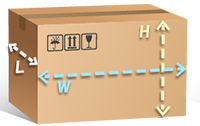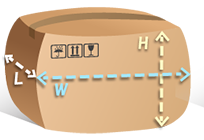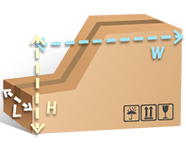#### Measurement and Calculation of Volume Weight

Volumetric weight is a calculation used to calculate the density of packages. Low-density packages typically take up more space than their actual weight.

For logistics routes that need to calculate the volumetric weight, we will compare the actual weight with the volumetric weight, and calculate the shipping cost based on the heavier one. The measurement method and calculation formula are stipulated by the logistics provider.

Warm reminder: usually the package will be squeezed by gravity during transportation, which will cause the carton to deform. The size data measured after receiving the package will have deviations. The size measured at the time of packaging shall prevail.

There are 3 ways to measure volume weight:

Standard package measurement methodthe package is a standard carton size, and the volume weight is calculated according to the length, width and height of the carton (as shown in the figure).For example: if the package is 15 in length, 30 in width and 20 in height through DHL channel, the volume weight = (15×30×20)/5000=1.8KG, and the actual weight is 1.2KG, then the shipping fee will be calculated based on the volume weight of 1.8KG .

Measurement method for curved surface parcels: If the parcel expands, deforms and bulges due to cargo extrusion, it shall be calculated based on the longest side of the parcel (as shown in the figure).For example: such as the DHL channel, the actual length of the carton is 15, the width is 30, and the height is 20; due to the extrusion of the goods, the length after sealing is 18, the width is 33, and the height is 22.

Then the volume weight = (18×33×22)/5000=2.7KG, and the actual weight is 2KG, then the shipping fee will be calculated based on the volume weight of 2.7KG.

Measurement method for irregular parcels: due to the actual size of the goods that need to be packed into irregular parcels, the volume weight of the parcels will be calculated according to the longest side (as shown in the figure).For example: such as the DHL channel, the longest length of irregular packages is 15, the longest width is 40, and the longest height is 25

Then volume weight = (15×40×25)/5000=3KG, and the actual weight is 2KG, then the shipping fee will be calculated based on the volume weight of 3KG.

#### What is volumetric weight?

Volumetric weight is a calculation used to calculate the density of packages. Low-density packages typically take up more space than their actual weight. After calculating the volumetric weight, compare it with the actual weight of the package, whichever is heavier will be used to calculate the shipping cost.